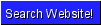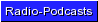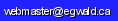Egwald Mathematics: Operations Research Linear Programming by Elmer G. Wiens Egwald's popular web pages are provided without cost to users. Please show your support by joining Egwald Web Services as a Facebook Fan:Follow Elmer Wiens on Twitter:A. Linear Programming.       1. Step through a linear programming graphical example. Then solve a problem by hand using the simplex algorithm, or the dual simplex algorithm.       2. Use either the primal simplex tableaux generator, or the dual simplex tableaux generator, to set up and solve your own linear programming problem, with a user-friendly interface.       3. Perform a sensitivity and/or postoptimal analysis of the solution to the data of an economics application of linear programming, with a user-friendly interface.             a. The profit maximization decision for a firm.             b. The least cost formula decision for a firm.       4. Step through a linear programming example using the online linear programming solver.       5. Use the online linear programming solver to solve your own L.P. problem.       6. As an application of linear programming, solve a zero sum two person game.       7. Try your skill on these sample problems.       8. A few linear programming references.   B. Practical use of linear programming.       1. Linear programming is used in the oligopoly / public firm model to derive the bonus rates for the managers of a public firm. See the derivation of the oligopoly/public firm model.   C. Game Theory. Return to the Operations Research home page.Copyright © Elmer G. Wiens:   Egwald Web ServicesAll Rights Reserved.    Inquiries